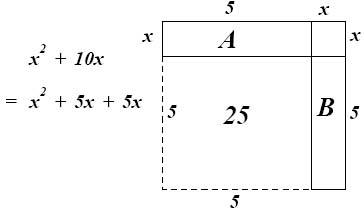# Intermediate Algebra Syllabus Spring '10

INSTRUCTOR:  Geoff Hagopian
Email: ghagopian@collegeofthedesert.edu
WEB SITE: http://geofhagopian.net
PHONE: 776-7223

#COURSE DESCRIPTION: The focus of this class is on solving problems using mathematical models involving basic linear, quadratic and exponential relations with an introduction to the concepts of functions.

In this course you will solve linear, quadratic and exponential equations and graph the solution sets of such equations in the coordinate plane. You will solve systems of linear equations, learn to manipulate rational exponents, solve quadratic inequalities, apply the distance and midpoint formulas, examine standard forms for the equation of a circle and model exponential growth/decay.

The "Rule of Three" is a general principle that governs our methods in this course.
This rule asserts that a good way to solve problems in mathematics is through the analysis of

(1) tabulated data (rows and columns of correlated numbers)
(2) visual displays (graphs)
(3) equations relating variables (for instance, x2 + y2 = 7xy.)

The idea is that the problems we study are best understood after using all of these approaches.

Topics include

• systems of linear and quadratic equations and inequalities in several variables
• functions: notation and classification
• powers, roots and polynomials functions
• exponential/logarithmic functions
• circles, parabolas and other conic sections

TEXTBOOK: Intermediate Algebra, Functions, and Graphs by Yoshiwara.
This text emphasizes basic algebra and the Rule of Three in mathematical modeling and problem solving. The assigned reading and problems are crucial and it will be obvious that you haven’t done it if you are not prepared with questions about these in class.

We won't be able to cover every problem type during class, but you will be responsible for everything discussed in the text, and to create novel solutions to some problems. If there is something you don’t understand, it’s up to you to be aware of that and to ask questions to get beyond the various confusions that are normal in the learning process.

LECTURE/HOMEWORK/LABS:  Generally, you can expect the most important part of your learning to come from applying yourself to the problems assigned for homework and lab time.  Of course, you will have a much easier time doing homework and performing well on exams if you pay attention and take good notes during lecture. Complete the homework assignment for each section to be well prepared for quizzes and exams. Do assignments to the best of your ability before the next class and have specific questions about places where you become stuck or confused.  You should be prepared at the beginning of each class with questions from the homework.

Note: You will also need to log onto the WebAssign homework system and purchase this service as . You will be responsible for keeping a homework notebook that can be presented on demand, but you will also need to log onto the WebAssign homework system at www.webassign.com and complete regular assignments there.

EXAMS:  Seven chapter tests (one after each chapter) and a cumulative final exam. This means a significant exam every two weeks or so. Keep in mind that the point of these exams is to test your understanding of the concepts covered; that is, they will not necessarily consist of just homework problems with the numbers changed, but may involve novel problems testing your understanding of how to use basic principles of algebra in context.

CALCULATORS:  A scientific calculator is required for this course. Though the text book devotes a large amount of material to using a graphing calculator, a graphing calculator is not required for this class.  Whenever textbook problems request the use a graphing calculator (as is often the case), pencil and paper is all you will need.

ATTENDANCE:  You are required to attend all lecture and lab sessions: 6 hours per week for 16 weeks: a total of 96 hours.  The two most significant indicators for success in this course are attendance and completion of the homework. If you're not there doing what it is that defines the class, you missed it.

GRADE:  Your grade in this class will be a weighted average of your attendance, homework quiz, chapter test and final exam scores, using the following apportionment:
Attendance/quiz: 10 pts.
Homework: 20 pts.
Chapter tests: 50 pts.
Final Exam: 20 pts.